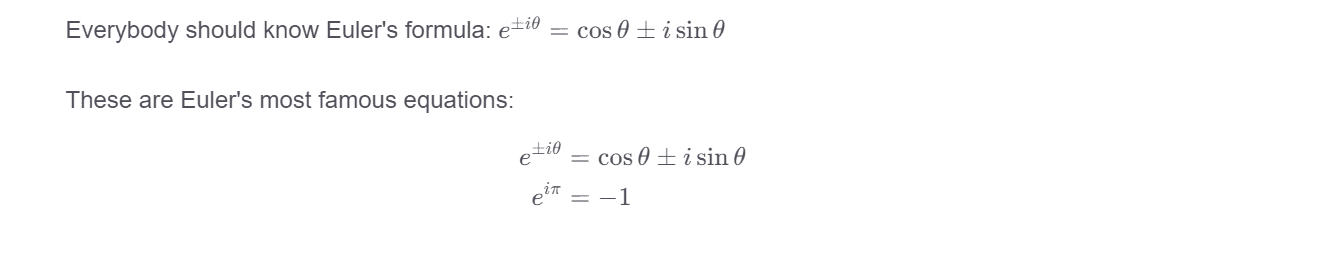# Math¶

Zettlr supports writing math and formulae using the KaTeX library. In order to use math, enclose your formulae with dollar signs ($...$). You can also use so-called displaymath blocks by using two dollar signs ($$...$$) instead of a single dollar sign to enclose your formulae. Anything detected as inline math or displaymath block will receive proper MathTeX syntax highlighting.

Everybody should know Euler's formula: $e^{ \pm i\theta } = \cos \theta \pm i\sin \theta$

These are Euler's most famous equations:
\begin{aligned} e^{ \pm i\theta } & = \cos \theta \pm i\sin \theta \\ e^{i \pi} & = -1 \end{aligned}


To prevent confusion with amounts of money, spaces between the formula and the dollar signs are not allowed!

If you activate the corresponding option, Zettlr will immediately render formulae in place, making it easy for you to proofread what you typed and fix errors. If a formula contains an error, this will be shown by Zettlr. The above example is rendered like this:For an overview of which functions and symbols you can use with the KaTex library, you can have a look on their documentation: https://katex.org/docs/supported.html